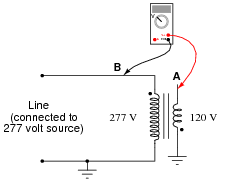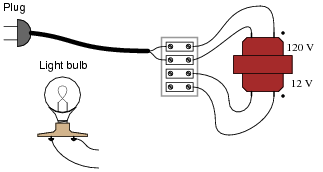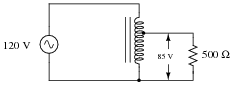# Autotransformers

## AC Electric Circuits

• #### Question 1

How much voltage is there between the following pairs of points in this circuit?Between C and F: VCF =
Between A and B: VAB =
Between D and E: VDE =
Between D and F: VDF =
• #### Question 2

How much voltage is there between the following pairs of points in this circuit?Between D and F: VDF =
Between A and B: VAB =
Between D and E: VDE =
Between C and F: VCF =
• #### Question 3

How much AC voltage is there between points A and B in this circuit?• #### Question 4

Connect the windings of this step-down transformer so that it “boosts” the voltage going to the load by 12 volts:• #### Question 5

Connect the windings of this step-down transformer so that it “bucks” the voltage going to the light bulb by 12 volts:• #### Question 6

How could we possibly determine the phasing of the windings in this step-down transformer (120 VAC, 60 Hz primary) so we would know how to connect them for either boosting or bucking service?• #### Question 7

Suppose you had an audio impedance-matching transformer (1000 Ω to 8 Ω), and you wished to know the phase relationships of the windings. Unfortunately, the only test equipment you have is an analog DC voltmeter and a 9-volt battery. Explain how you would use these two devices to test winding “polarity.”Note: you may disregard the center-tap of the 1000 Ω primary winding.

• #### Question 8

It should be obvious that the load voltage in this resistive voltage divider circuit will be less than 30 volts AC (RMS):Likewise, it should be apparent that the load voltage in this inductive voltage divider circuit will be less than 30 volts AC (RMS):From this brief analysis it would appear these two voltage divider circuits are equivalent to one another. However, there is a very important difference in terms of current: load versus supply. Estimate the load and source currents in each of these divider circuits, and comment on the difference(s) between the two.

• #### Question 9

A popular piece of electrical bench test equipment is the Variac. Explain what a Variac is, how it works, and what applications it might be used for.

• #### Question 10

Devices called step regulators are sometimes used in electrical power distribution systems to boost or suppress line voltages, typically to a maximum of 10%:The “Line” side of this autotransformer is the source of power, and the “Load” side connects to those devices consuming electrical power. The “Reversing switch” alters the transformer’s function from boost to buck.

Determine which switch position is “boost” and which is “buck,” and then determine which way the moving contact (the arrow symbol) needs to be moved in order to increase the voltage output, and to decrease the voltage output.

• #### Question 11

Calculate all currents in this autotransformer circuit, assuming perfect (100%) efficiency:• #### Question 12

Suppose the load in this circuit suddenly stops receiving power:Your first step in troubleshooting is to take some voltage measurements:

VAB = 120 V
VEC = 0 V
VDC = 120 V

Based on these measurements, where do you think the fault is in this circuit, and what type of fault is it?